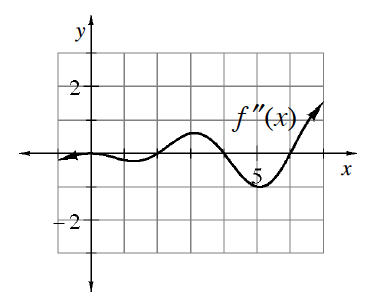### Home > APCALC > Chapter 9 > Lesson 9.2.1 > Problem9-52

9-52.

The graph at right shows $y = f^{\prime\prime}(x)$. Use it to answer the following questions.

1. Over what interval(s) is $f$ concave up?

$f$ is concave up when $f ^{\prime\prime}$ is positive. Where is the given graph positive?

1. Estimate $f^{\prime\prime\prime}(5)$.

This is asking for the slope of the given graph at $x = 5$.

1. At what $x$-value(s) does $f$ have a point of inflection?

This is where $f ^{\prime\prime}$ changes sign. Where does the given graph change from negative to positive and vice versa?

1. At what $x$-value(s) does $f^\prime$ have a minimum valu​e?

$f^\prime$ has a minimum where its slope changes from negative to positive.
The slope of $f^\prime$ is $f ^{\prime\prime}$. So where does $f ^{\prime\prime}$ change from negative to positive?